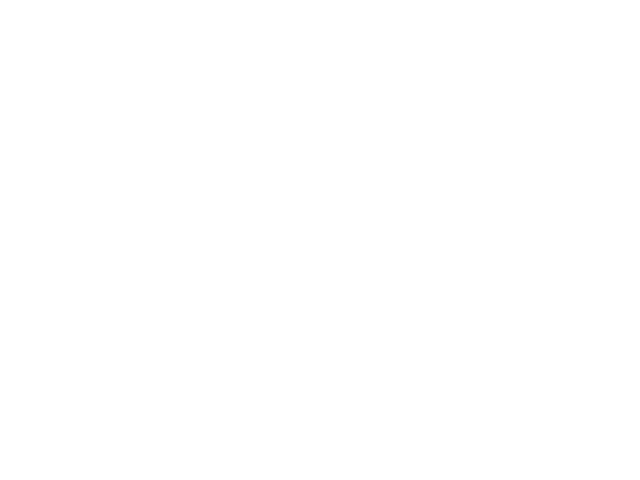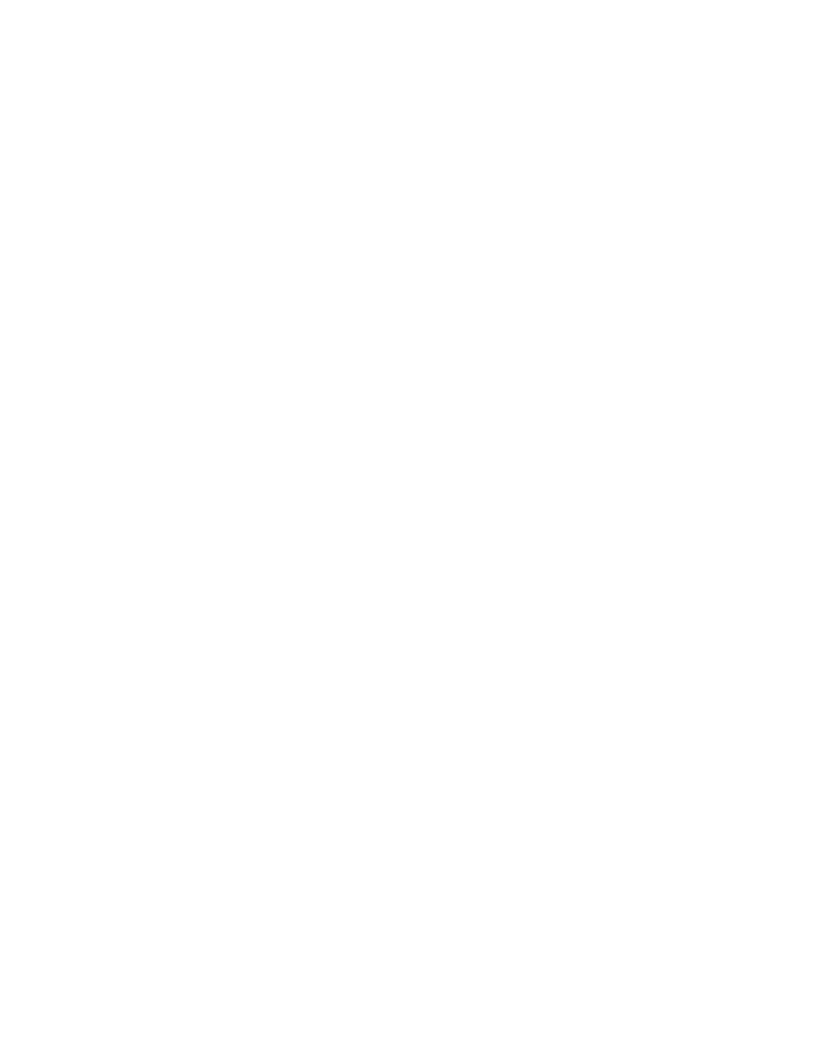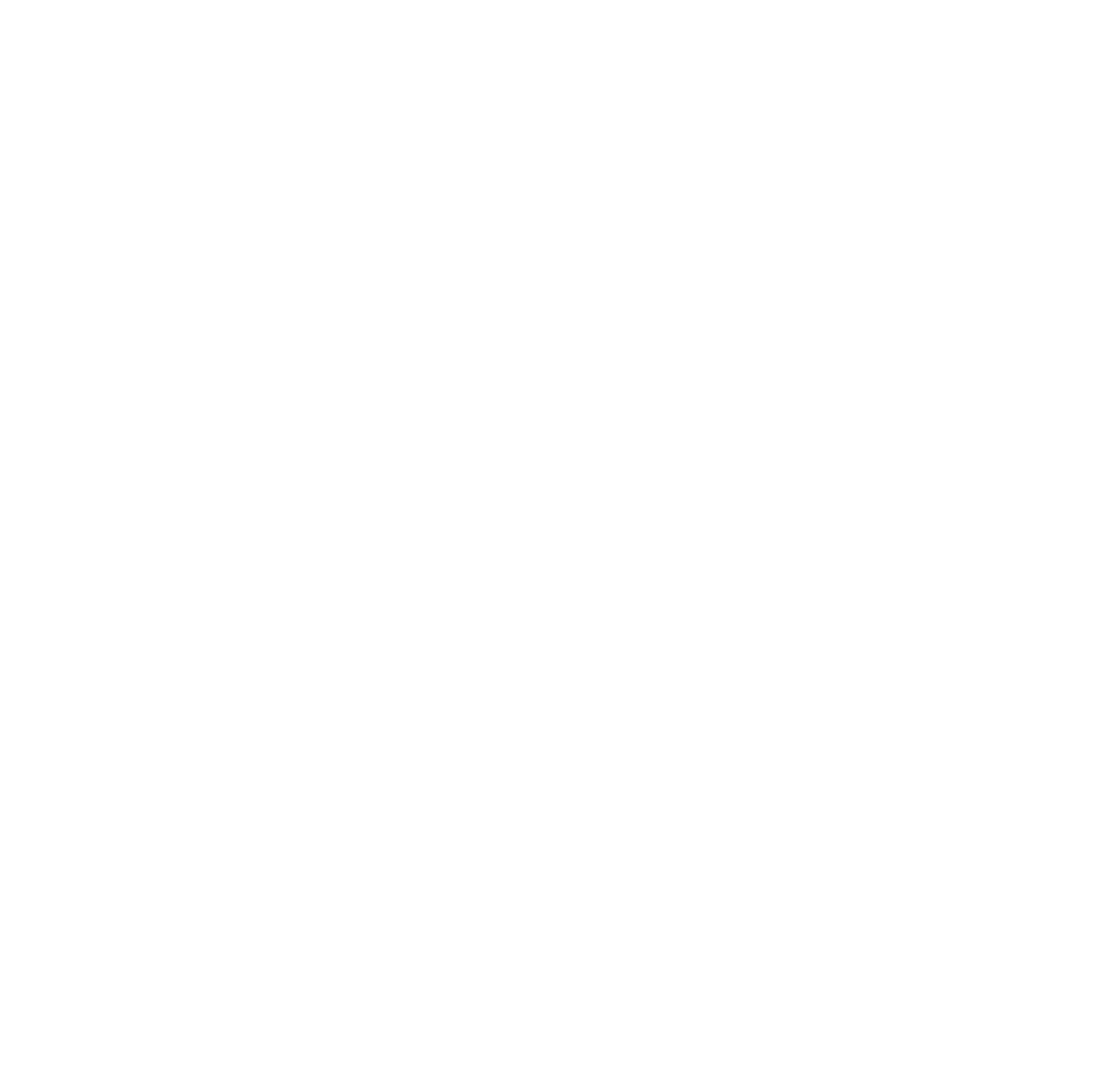## What do you notice?

I want you to think about the following even/odd numbers, patterns, multiples, factors, multiplication, common multiples, prime/composite numbers.

# Triangular Numbers

• Students in each group should work to build triangles of different sizes.
• After building a triangle the group writes down the number of counters that make up each triangle. Each of these numbers is a Triangular Number.
• As each group builds triangles they should fill in the missing blanks on their worksheet with the correct number of dots for the given triangle.
• Groups will fill out the worksheet as they build triangles.